1668225280

# Instructions to Take A Browser Screenshot In Laravel | For Beginners

This tutorial will give you an example of laravel take screenshot. Here you will learn laravel take screenshot of website url. you can understand a concept of how to take website screenshot from url in laravel. we will help you to give an example of how to take a screenshot in laravel. Alright, let us dive into the details.

You can use this example with laravel 6, laravel 7, laravel 8 and laravel 9 versions.

We will use spatie/browsershot composer package for take a screenshot of website in laravel. we will use url(), setOption(), windowSize(), waitUntilNetworkIdle() and save() method to capture browser screenshot in laravel. so let's follow the below steps:

Step 1 : Install Laravel

first of all we need to get fresh Laravel version application using bellow command, So open your terminal OR command prompt and run bellow command:

``composer create-project laravel/laravel example-app``

Step 2: Install spatie/browsershot Package

here, we will install spatie/browsershot package for take a screenshot of url in laravel. so, let's run the bellow commands:

``composer require spatie/browsershot``

Next, we need to install puppeteer npm package, that used to capture screenshot. let's installed using the below command:

``npm install puppeteer --global``

Step 3: Create Route

In this is step we need to create one route for capture browser screenshot. let's add the below route on web.php file.

routes/web.php

``````<?php

use App\Http\Controllers\DemoController;

/*
|--------------------------------------------------------------------------
| Web Routes
|--------------------------------------------------------------------------
|
| Here is where you can register web routes for your application. These
| routes are loaded by the RouteServiceProvider within a group which
| contains the "web" middleware group. Now create something great!
|
*/

Route::get('demo', [DemoController::class,'index']);``````

Step 4: Create Controller

in this step, we need to create DemoController with index()method.

Add the below code on controller file.

app/Http/Controllers/DemoController.php

``````<?php

namespace App\Http\Controllers;

use Illuminate\Http\Request;
use Spatie\Browsershot\Browsershot;

class DemoController extends Controller
{
/**
* Write code on Method
*
* @return response()
*/
public function index(Request \$request)
{
Browsershot::url('https://www.itsolutionstuff.com')
->setOption('landscape', true)
->windowSize(3840, 2160)
->waitUntilNetworkIdle()
->save('itsolutionstuff.jpg');

dd("Done");
}
}``````

Run Laravel App:

All the required steps have been done, now you have to type the given below command and hit enter to run the Laravel app:

``php artisan serve``

``http://localhost:8000/demo``

Original article source at: https://www.itsolutionstuff.com

## Buddha Community1672928580

## How to Bash Read Command

Bash has no built-in function to take the user’s input from the terminal. The read command of Bash is used to take the user’s input from the terminal. This command has different options to take an input from the user in different ways. Multiple inputs can be taken using the single read command. Different ways of using this command in the Bash script are described in this tutorial.

## Syntax

The read command can be used without any argument or option. Many types of options can be used with this command to take the input of the particular data type. It can take more input from the user by defining the multiple variables with this command.

## Some Useful Options of the Read Command

Some options of the read command require an additional parameter to use. The most commonly used options of the read command are mentioned in the following:

## Different Examples of the Read Command

The uses of read command with different options are shown in this part of this tutorial.

Example 1: Using Read Command without Any Option and variable

Create a Bash file with the following script that takes the input from the terminal using the read command without any option and variable. If no variable is used with the read command, the input value is stored in the \$REPLY variable. The value of this variable is printed later after taking the input.

``````#!/bin/bash
#Print the prompt message
echo "Enter your favorite color: "
#Take the input
#Print the input value

Output:

The following output appears if the “Blue” value is taken as an input:Example 2: Using Read Command with a Variable

Create a Bash file with the following script that takes the input from the terminal using the read command with a variable. The method of taking the single or multiple variables using a read command is shown in this example. The values of all variables are printed later.

``````#!/bin/bash
#Print the prompt message
echo "Enter the product name: "
#Take the input with a single variable

#Print the prompt message
echo "Enter the color variations of the product: "
#Take three input values in three variables

#Print the input value
echo "The product name is \$item."
#Print the input values
echo "Available colors are \$color1, \$color2, and \$color3."``````

Output:

The following output appears after taking a single input first and three inputs later: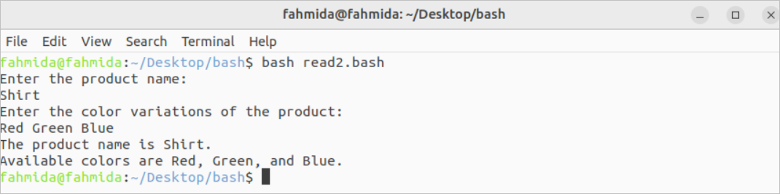Example 3: Using Read Command with -p Option

Create a Bash file with the following script that takes the input from the terminal using the read command with a variable and the -p option. The input value is printed later.

``````#!/bin/bash
#Take the input with the prompt message
read -p "Enter the book name: " book
#Print the input value
echo "Book name: \$book"``````

Output:

The following output appears after taking the input: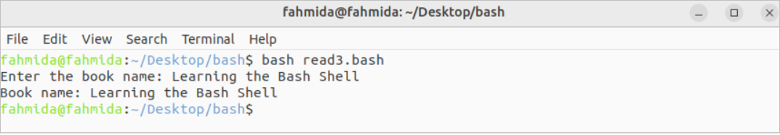Example 4: Using Read Command with -s Option

Create a Bash file with the following script that takes the input from the terminal using the read command with a variable and the -s option. The input value of the password will not be displayed for the -s option. The input values are checked later for authentication. A success or failure message is also printed.

``````#!/bin/bash
#Take the input with the prompt message
#Take the secret input with the prompt message

echo ""

#Check the email and password for authentication
then
#Print the success message
echo "Authenticated."
else
#Print the failure message
echo "Not authenticated."
fi``````

Output:

The following output appears after taking the valid and invalid input values: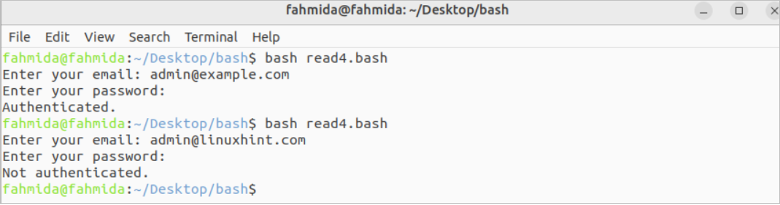Example 5: Using Read Command with -a Option

Create a Bash file with the following script that takes the input from the terminal using the read command with a variable and the -a option. The array values are printed later after taking the input values from the terminal.

``````#!/bin/bash
echo "Enter the country names: "
#Take multiple inputs using an array

echo "Country names are:"
for country in \${countries[@]}
do
echo \$country
done``````

Output:

The following output appears after taking the array values: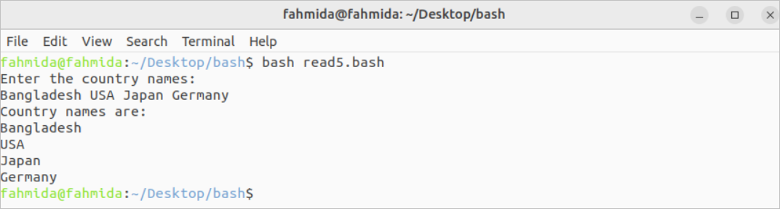Example 6: Using Read Command with -n Option

Create a Bash file with the following script that takes the input from the terminal using the read command with a variable and the -n option.

``````#!/bin/bash
#Print the prompt message
echo "Enter the product code: "
#Take the input of five characters
echo ""
#Print the input value
echo "The product code is \$code"``````

Output:

The following output appears if the “78342” value is taken as input: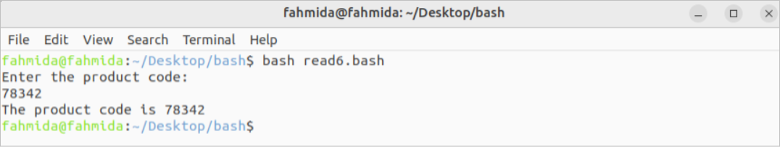Example 7: Using Read Command with -t Option

Create a Bash file with the following script that takes the input from the terminal using the read command with a variable and the -t option.

``````#!/bin/bash
#Print the prompt message
echo -n "Write the result of 10-6: "
#Take the input of five characters

#Check the input value
if [[ \$answer == "4" ]]
then
else
fi``````

Output:

The following output appears after taking the correct and incorrect input values: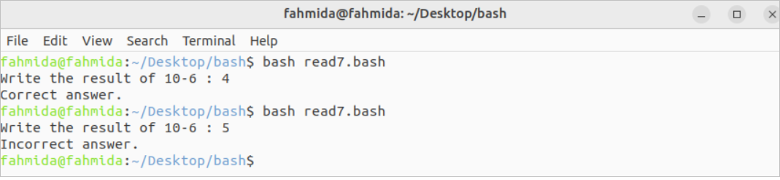## Conclusion

The uses of some useful options of the read command are explained in this tutorial using multiple examples to know the basic uses of the read command.

Original article source at: https://linuxhint.com/

1637592240

## In diesem Python-Beitrag erfahren Sie Folgendes:

• Was ist eine lineare Suche?
• Linearer Suchalgorithmus
• Schreiben Sie ein Python-Programm für die lineare Suche mit While-Schleife
• Schreiben Sie ein Python-Programm für die lineare Suche mit der For-Schleife
• Lineare Suche im Python-Programm mit Rekursion

### Was ist eine lineare Suche?

Eine lineare Suche, auch bekannt als sequentielle Suche, diese Methode wird verwendet, um ein Element innerhalb einer Liste oder eines Arrays zu finden. Es überprüft jedes Element der Liste nacheinander / sequentiell, bis eine Übereinstimmung gefunden wird oder die gesamte Liste durchsucht wurde.

### Linearer Suchalgorithmus

Implementieren Sie die lineare Suche mit den folgenden Schritten:

• Durchlaufen Sie die Liste/das Array mit einer Schleife.
• Verknüpfen Sie in jeder Iteration den  `target` Wert mit dem angegebenen Wert der Liste/des Arrays.
• Wenn die Werte übereinstimmen, geben Sie den aktuellen Index der Liste/des Arrays zurück.
• Fahren Sie andernfalls mit dem nächsten Array-/Listenelement fort.
• Wenn keine Übereinstimmung gefunden wird, geben Sie zurück  `-1`.

### Schreiben Sie ein Python-Programm für die lineare Suche mit While-Schleife

``````# python program for linear search using while loop

#define list
lst = []

#take input list size
num = int(input("Enter size of list :- "))

for n in range(num):
#append element in list/array
numbers = int(input("Enter the array of %d element :- " %n))
lst.append(numbers)

#take input number to be find in list
x = int(input("Enter number to search in list :- "))

i = 0
flag = False

while i < len(lst):
if lst[i] == x:
flag = True
break

i = i + 1

if flag == 1:
print('{} was found at index {}.'.format(x, i))
else:
``````

Nach der Ausführung des Programms lautet die Ausgabe:

``````Enter size of list :-  5
Enter the array of 0 element :-  10
Enter the array of 1 element :-  23
Enter the array of 2 element :-  56
Enter the array of 3 element :-  89
Enter the array of 4 element :-  200
Enter number to search in list :-  89
89 was found at index 3.
``````

### Schreiben Sie ein Python-Programm für die lineare Suche mit der For-Schleife

``````# python program for linear search using for loop

#define list
lst = []

#take input list size
num = int(input("Enter size of list :- "))

for n in range(num):
#append element in list/array
numbers = int(input("Enter the array of %d element :- " %n))
lst.append(numbers)

#take input number to be find in list
x = int(input("Enter number to search in list :- "))

i = 0
flag = False

for i in range(len(lst)):
if lst[i] == x:
flag = True
break

if flag == 1:
print('{} was found at index {}.'.format(x, i))
else:

``````

Nach der Ausführung des Programms lautet die Ausgabe:

``````Enter size of list :-  6
Enter the array of 0 element :-  25
Enter the array of 1 element :-  50
Enter the array of 2 element :-  100
Enter the array of 3 element :-  200
Enter the array of 4 element :-  250
Enter the array of 5 element :-  650
Enter number to search in list :-  200
200 was found at index 3.

``````

### Lineare Suche im Python-Programm mit Rekursion

``````# python program for linear search using for loop

#define list
lst = []

#take input list size
num = int(input("Enter size of list :- "))

for n in range(num):
#append element in list/array
numbers = int(input("Enter the array of %d element :- " %n))
lst.append(numbers)

#take input number to be find in list
x = int(input("Enter number to search in list :- "))

# Recursive function to linear search x in arr[l..r]
def recLinearSearch( arr, l, r, x):
if r < l:
return -1
if arr[l] == x:
return l
if arr[r] == x:
return r
return recLinearSearch(arr, l+1, r-1, x)

res = recLinearSearch(lst, 0, len(lst)-1, x)

if res != -1:
print('{} was found at index {}.'.format(x, res))
else:

``````

Nach der Ausführung des Programms lautet die Ausgabe:

``````Enter size of list :-  5
Enter the array of 0 element :-  14
Enter the array of 1 element :-  25
Enter the array of 2 element :-  63
Enter the array of 3 element :-  42
Enter the array of 4 element :-  78
Enter number to search in list :-  78
78 was found at index 4.``````1637563159

## Trong bài đăng python này, bạn sẽ tìm hiểu những điều sau:

• Tìm kiếm tuyến tính là gì?
• Thuật toán tìm kiếm tuyến tính
• Viết chương trình Python để tìm kiếm tuyến tính bằng While Loop
• Viết chương trình Python để tìm kiếm tuyến tính bằng For Loop
• Tìm kiếm tuyến tính trong Chương trình Python sử dụng Đệ quy

### Tìm kiếm tuyến tính là gì?

Trước hết, Tìm kiếm tuyến tính, còn được gọi là tìm kiếm tuần tự, phương pháp này được sử dụng để tìm một phần tử trong danh sách hoặc mảng. Nó kiểm tra từng phần tử của danh sách một / tuần tự cho đến khi tìm thấy một kết quả phù hợp hoặc toàn bộ danh sách đã được tìm kiếm.

### Thuật toán tìm kiếm tuyến tính

Triển khai tìm kiếm tuyến tính theo các bước sau:

• Duyệt qua danh sách / mảng bằng cách sử dụng một vòng lặp.
• Trong mỗi lần lặp, hãy liên kết  `target` giá trị với giá trị đã cho của danh sách / mảng.
• Nếu các giá trị khớp, trả về chỉ mục hiện tại của danh sách / mảng.
• Nếu không, hãy chuyển sang phần tử mảng / danh sách tiếp theo.
• Nếu không tìm thấy kết quả phù hợp, hãy quay lại  `-1`.

### Viết chương trình Python để tìm kiếm tuyến tính bằng While Loop

``````# python program for linear search using while loop

#define list
lst = []

#take input list size
num = int(input("Enter size of list :- "))

for n in range(num):
#append element in list/array
numbers = int(input("Enter the array of %d element :- " %n))
lst.append(numbers)

#take input number to be find in list
x = int(input("Enter number to search in list :- "))

i = 0
flag = False

while i < len(lst):
if lst[i] == x:
flag = True
break

i = i + 1

if flag == 1:
print('{} was found at index {}.'.format(x, i))
else:
``````

Sau khi thực hiện chương trình, kết quả đầu ra sẽ là:

``````Enter size of list :-  5
Enter the array of 0 element :-  10
Enter the array of 1 element :-  23
Enter the array of 2 element :-  56
Enter the array of 3 element :-  89
Enter the array of 4 element :-  200
Enter number to search in list :-  89
89 was found at index 3.
``````

### Viết chương trình Python để tìm kiếm tuyến tính bằng For Loop

``````# python program for linear search using for loop

#define list
lst = []

#take input list size
num = int(input("Enter size of list :- "))

for n in range(num):
#append element in list/array
numbers = int(input("Enter the array of %d element :- " %n))
lst.append(numbers)

#take input number to be find in list
x = int(input("Enter number to search in list :- "))

i = 0
flag = False

for i in range(len(lst)):
if lst[i] == x:
flag = True
break

if flag == 1:
print('{} was found at index {}.'.format(x, i))
else:

``````

Sau khi thực hiện chương trình, kết quả đầu ra sẽ là:

``````Enter size of list :-  6
Enter the array of 0 element :-  25
Enter the array of 1 element :-  50
Enter the array of 2 element :-  100
Enter the array of 3 element :-  200
Enter the array of 4 element :-  250
Enter the array of 5 element :-  650
Enter number to search in list :-  200
200 was found at index 3.

``````

### Tìm kiếm tuyến tính trong Chương trình Python sử dụng Đệ quy

``````# python program for linear search using for loop

#define list
lst = []

#take input list size
num = int(input("Enter size of list :- "))

for n in range(num):
#append element in list/array
numbers = int(input("Enter the array of %d element :- " %n))
lst.append(numbers)

#take input number to be find in list
x = int(input("Enter number to search in list :- "))

# Recursive function to linear search x in arr[l..r]
def recLinearSearch( arr, l, r, x):
if r < l:
return -1
if arr[l] == x:
return l
if arr[r] == x:
return r
return recLinearSearch(arr, l+1, r-1, x)

res = recLinearSearch(lst, 0, len(lst)-1, x)

if res != -1:
print('{} was found at index {}.'.format(x, res))
else:

``````

Sau khi thực hiện chương trình, kết quả đầu ra sẽ là:

``````Enter size of list :-  5
Enter the array of 0 element :-  14
Enter the array of 1 element :-  25
Enter the array of 2 element :-  63
Enter the array of 3 element :-  42
Enter the array of 4 element :-  78
Enter number to search in list :-  78
78 was found at index 4.``````1636296420

## 線形検索のためのPythonプログラム

このチュートリアルでは、Pythonで線形検索プログラムを作成する方法を学習します。

まず、線形検索（シーケンシャル検索とも呼ばれます）は、リストまたは配列内の要素を見つけるために使用されます。一致するものが見つかるか、リスト全体が検索されるまで、リストの各要素を1つずつ/順番にチェックします。

### 線形探索アルゴリズム

• ループを使用してリスト/配列をトラバースします。
• すべての反復で、`target` 値をリスト/配列の指定された値に関連付け ます。
• 値が一致する場合は、リスト/配列の現在のインデックスを返します。
• それ以外の場合は、次の配列/リスト要素に移動します。
• 一致するものが見つからない場合は、を返し `-1`ます。

## 線形検索のためのPythonプログラム

• whileループを使用した線形検索用のPythonプログラム
• Forループを使用した線形検索用のPythonプログラム
• 再帰を使用したPythonプログラムでの線形検索

### whileループを使用した線形検索用のPythonプログラム

``````# python program for linear search using while loop

#define list
lst = []

#take input list size
num = int(input("Enter size of list :- "))

for n in range(num):
#append element in list/array
numbers = int(input("Enter the array of %d element :- " %n))
lst.append(numbers)

#take input number to be find in list
x = int(input("Enter number to search in list :- "))

i = 0
flag = False

while i < len(lst):
if lst[i] == x:
flag = True
break

i = i + 1

if flag == 1:
print('{} was found at index {}.'.format(x, i))
else:
``````

プログラムの実行後、出力は次のようになります。

``````Enter size of list :-  5
Enter the array of 0 element :-  10
Enter the array of 1 element :-  23
Enter the array of 2 element :-  56
Enter the array of 3 element :-  89
Enter the array of 4 element :-  200
Enter number to search in list :-  89
89 was found at index 3.``````

### Forループを使用した線形検索用のPythonプログラム

``````# python program for linear search using for loop

#define list
lst = []

#take input list size
num = int(input("Enter size of list :- "))

for n in range(num):
#append element in list/array
numbers = int(input("Enter the array of %d element :- " %n))
lst.append(numbers)

#take input number to be find in list
x = int(input("Enter number to search in list :- "))

i = 0
flag = False

for i in range(len(lst)):
if lst[i] == x:
flag = True
break

if flag == 1:
print('{} was found at index {}.'.format(x, i))
else:
``````

プログラムの実行後、出力は次のようになります。

``````Enter size of list :-  6
Enter the array of 0 element :-  25
Enter the array of 1 element :-  50
Enter the array of 2 element :-  100
Enter the array of 3 element :-  200
Enter the array of 4 element :-  250
Enter the array of 5 element :-  650
Enter number to search in list :-  200
200 was found at index 3.``````

### 再帰を使用したPythonプログラムでの線形検索

``````# python program for linear search using for loop

#define list
lst = []

#take input list size
num = int(input("Enter size of list :- "))

for n in range(num):
#append element in list/array
numbers = int(input("Enter the array of %d element :- " %n))
lst.append(numbers)

#take input number to be find in list
x = int(input("Enter number to search in list :- "))

# Recursive function to linear search x in arr[l..r]
def recLinearSearch( arr, l, r, x):
if r < l:
return -1
if arr[l] == x:
return l
if arr[r] == x:
return r
return recLinearSearch(arr, l+1, r-1, x)

res = recLinearSearch(lst, 0, len(lst)-1, x)

if res != -1:
print('{} was found at index {}.'.format(x, res))
else:
``````

プログラムの実行後、出力は次のようになります。

``````Enter size of list :-  5
Enter the array of 0 element :-  14
Enter the array of 1 element :-  25
Enter the array of 2 element :-  63
Enter the array of 3 element :-  42
Enter the array of 4 element :-  78
Enter number to search in list :-  78
78 was found at index 4.``````

1637595900

## Nesta postagem python, você aprenderá o seguinte:

• O que é uma pesquisa linear?
• Algoritmo de pesquisa linear
• Escreva um programa Python para pesquisa linear usando loop while
• Escreva um programa Python para pesquisa linear usando o loop for
• Pesquisa linear no programa Python usando recursão

### O que é uma pesquisa linear?

Em primeiro lugar, uma pesquisa linear, também conhecida como pesquisa sequencial, este método é usado para localizar um elemento dentro de uma lista ou array. Ele verifica cada elemento da lista um por um / sequencialmente até que uma correspondência seja encontrada ou toda a lista tenha sido pesquisada.

### Algoritmo de pesquisa linear

Implemente a pesquisa linear seguindo as etapas abaixo:

• Percorra a lista / array usando um loop.
• Em cada iteração, associe o `target` valor ao  valor fornecido da lista / matriz.
• Se os valores corresponderem, retorne o índice atual da lista / matriz.
• Caso contrário, vá para o próximo elemento de array / lista.
• Se nenhuma correspondência for encontrada, retorne  `-1`.

### Escreva um programa Python para pesquisa linear usando loop while

``````# python program for linear search using while loop

#define list
lst = []

#take input list size
num = int(input("Enter size of list :- "))

for n in range(num):
#append element in list/array
numbers = int(input("Enter the array of %d element :- " %n))
lst.append(numbers)

#take input number to be find in list
x = int(input("Enter number to search in list :- "))

i = 0
flag = False

while i < len(lst):
if lst[i] == x:
flag = True
break

i = i + 1

if flag == 1:
print('{} was found at index {}.'.format(x, i))
else:
``````

Depois de executar o programa, a saída será:

``````Enter size of list :-  5
Enter the array of 0 element :-  10
Enter the array of 1 element :-  23
Enter the array of 2 element :-  56
Enter the array of 3 element :-  89
Enter the array of 4 element :-  200
Enter number to search in list :-  89
89 was found at index 3.
``````

### Escreva um programa Python para pesquisa linear usando o loop for

``````# python program for linear search using for loop

#define list
lst = []

#take input list size
num = int(input("Enter size of list :- "))

for n in range(num):
#append element in list/array
numbers = int(input("Enter the array of %d element :- " %n))
lst.append(numbers)

#take input number to be find in list
x = int(input("Enter number to search in list :- "))

i = 0
flag = False

for i in range(len(lst)):
if lst[i] == x:
flag = True
break

if flag == 1:
print('{} was found at index {}.'.format(x, i))
else:

``````

Depois de executar o programa, a saída será:

``````Enter size of list :-  6
Enter the array of 0 element :-  25
Enter the array of 1 element :-  50
Enter the array of 2 element :-  100
Enter the array of 3 element :-  200
Enter the array of 4 element :-  250
Enter the array of 5 element :-  650
Enter number to search in list :-  200
200 was found at index 3.

``````

### Pesquisa linear no programa Python usando recursão

``````# python program for linear search using for loop

#define list
lst = []

#take input list size
num = int(input("Enter size of list :- "))

for n in range(num):
#append element in list/array
numbers = int(input("Enter the array of %d element :- " %n))
lst.append(numbers)

#take input number to be find in list
x = int(input("Enter number to search in list :- "))

# Recursive function to linear search x in arr[l..r]
def recLinearSearch( arr, l, r, x):
if r < l:
return -1
if arr[l] == x:
return l
if arr[r] == x:
return r
return recLinearSearch(arr, l+1, r-1, x)

res = recLinearSearch(lst, 0, len(lst)-1, x)

if res != -1:
print('{} was found at index {}.'.format(x, res))
else:
``````Enter size of list :-  5